Publicité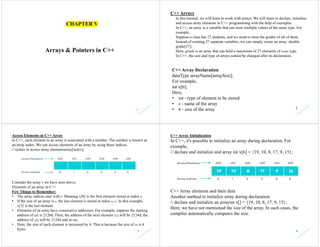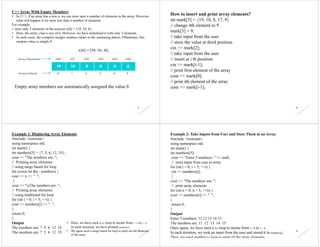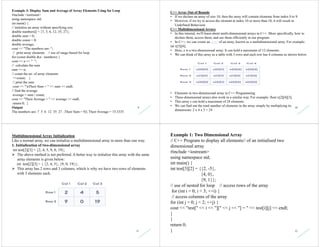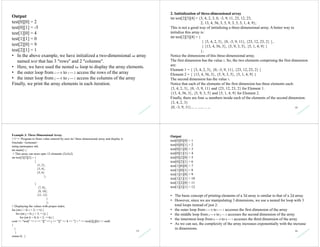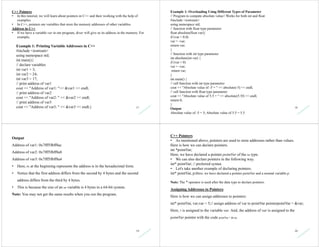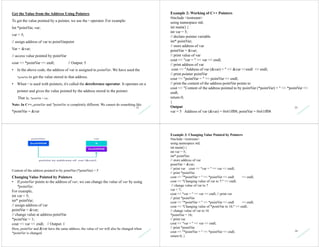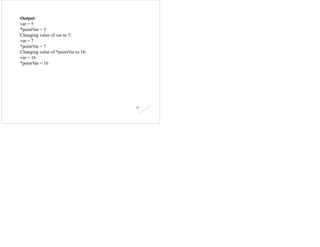1 sur 7
Publicité

### Chap 6 c++

1. 1 Arrays & Pointers in C++ CHAPTER V 2 C++ Arrays In this tutorial, we will learn to work with arrays. We will learn to declare, initialize, and access array elements in C++ programming with the help of examples. In C++, an array is a variable that can store multiple values of the same type. For example, Suppose a class has 27 students, and we need to store the grades of all of them. Instead of creating 27 separate variables, we can simply create an array: double grade; Here, grade is an array that can hold a maximum of 27 elements of double type. In C++, the size and type of arrays cannot be changed after its declaration. C++ Array Declaration dataType arrayName[arraySize]; For example, int x; Here, • int - type of element to be stored • x - name of the array • 6 - size of the array 3 Access Elements in C++ Array In C++, each element in an array is associated with a number. The number is known as an array index. We can access elements of an array by using those indices. // syntax to access array elementsarray[index]; Consider the array x we have seen above. Elements of an array in C++ Few Things to Remember: • The array indices start with 0. Meaning x is the first element stored at index 0. • If the size of an array is n, the last element is stored at index (n-1). In this example, x is the last element. • Elements of an array have consecutive addresses. For example, suppose the starting address of x is 2120d. Then, the address of the next element x will be 2124d, the address of x will be 2128d and so on. • Here, the size of each element is increased by 4. This is because the size of int is 4 bytes. 4 C++ Array Initialization In C++, it's possible to initialize an array during declaration. For example, // declare and initialize and array int x = {19, 10, 8, 17, 9, 15}; C++ Array elements and their data Another method to initialize array during declaration: // declare and initialize an arrayint x[] = {19, 10, 8, 17, 9, 15}; Here, we have not mentioned the size of the array. In such cases, the compiler automatically computes the size.
2. 5 C++ Array With Empty Members • In C++, if an array has a size n, we can store upto n number of elements in the array. However, what will happen if we store less than n number of elements. For example, // store only 3 elements in the arrayint x = {19, 10, 8}; • Here, the array x has a size of 6. However, we have initialized it with only 3 elements. • In such cases, the compiler assigns random values to the remaining places. Oftentimes, this random value is simply 0 Empty array members are automatically assigned the value 0 6 How to insert and print array elements? int mark = {19, 10, 8, 17, 9} // change 4th element to 9 mark = 9; // take input from the user // store the value at third position cin >> mark; // take input from the user // insert at i th position cin >> mark[i-1]; // print first element of the array cout << mark; // print ith element of the array cout >> mark[i-1]; 7 Example 1: Displaying Array Elements #include <iostream> using namespace std; int main() { int numbers = {7, 5, 6, 12, 35}; cout << "The numbers are: "; // Printing array elements // using range based for loop for (const int &n : numbers) { cout << n << " "; } cout << "nThe numbers are: "; // Printing array elements // using traditional for loop for (int i = 0; i < 5; ++i) { cout << numbers[i] << " "; } return 0; } Output The numbers are: 7 5 6 12 35 The numbers are: 7 5 6 12 35 • Here, we have used a for loop to iterate from i = 0 to i = 4. In each iteration, we have printed numbers[i]. • We again used a range based for loop to print out the elements of the array. 8 Example 2: Take Inputs from User and Store Them in an Array #include <iostream> using namespace std; int main() { int numbers; cout << "Enter 5 numbers: " << endl; // store input from user to array for (int i = 0; i < 5; ++i) { cin >> numbers[i]; } cout << "The numbers are: "; // print array elements for (int n = 0; n < 5; ++n) { cout << numbers[n] << " "; } return 0; } Output Enter 5 numbers: 11 12 13 14 15 The numbers are: 11 12 13 14 15 Once again, we have used a for loop to iterate from i = 0 to i = 4. In each iteration, we took an input from the user and stored it in numbers[i]. Then, we used another for loop to print all the array elements.
3. 9 Example 3: Display Sum and Average of Array Elements Using for Loop #include <iostream> using namespace std; int main() { // initialize an array without specifying size double numbers[] = {7, 5, 6, 12, 35, 27}; double sum = 0; double count = 0; double average; cout << "The numbers are: "; // print array elements // use of range-based for loop for (const double &n : numbers) { cout << n << " "; // calculate the sum sum += n; // count the no. of array elements ++count; } // print the sum cout << "nTheir Sum = " << sum << endl; // find the average average = sum / count; cout << "Their Average = " << average << endl; return 0; } Output The numbers are: 7 5 6 12 35 27 ;Their Sum = 92; Their Average = 15.3333 10 C++ Array Out of Bounds • If we declare an array of size 10, then the array will contain elements from index 0 to 9. • However, if we try to access the element at index 10 or more than 10, it will result in Undefined Behaviour. C++ Multidimensional Arrays • In this tutorial, we'll learn about multi-dimensional arrays in C++. More specifically, how to declare them, access them, and use them efficiently in our program. • In C++, we can create an array of an array, known as a multidimensional array. For example: int x; • Here, x is a two-dimensional array. It can hold a maximum of 12 elements. • We can think of this array as a table with 3 rows and each row has 4 columns as shown below. • Elements in two-dimensional array in C++ Programming • Three-dimensional arrays also work in a similar way. For example: float x; • This array x can hold a maximum of 24 elements. • We can find out the total number of elements in the array simply by multiplying its dimensions: 2 x 4 x 3 = 24 11 Multidimensional Array Initialization Like a normal array, we can initialize a multidimensional array in more than one way. 1. Initialization of two-dimensional array int test = {2, 4, 5, 9, 0, 19};  The above method is not preferred. A better way to initialize this array with the same array elements is given below: int test = { {2, 4, 5}, {9, 0, 19}};  This array has 2 rows and 3 columns, which is why we have two rows of elements with 3 elements each. 12 Example 1: Two Dimensional Array // C++ Program to display all elements// of an initialised two dimensional array #include <iostream> using namespace std; int main() { int test = {{2, -5}, {4, 0}, {9, 1}}; // use of nested for loop // access rows of the array for (int i = 0; i < 3; ++i) { // access columns of the array for (int j = 0; j < 2; ++j) { cout << "test[" << i << "][" << j << "] = " << test[i][j] << endl; } } return 0; }
4. Output test = 2 test = -5 test = 4 test = 0 test = 9 test = 1 • In the above example, we have initialized a two-dimensional int array named test that has 3 "rows" and 2 "columns". • Here, we have used the nested for loop to display the array elements. • the outer loop from i == 0 to i == 2 access the rows of the array • the inner loop from j == 0 to j == 1 access the columns of the array Finally, we print the array elements in each iteration. 14 2. Initialization of three-dimensional array int test = {3, 4, 2, 3, 0, -3, 9, 11, 23, 12, 23, 2, 13, 4, 56, 3, 5, 9, 3, 5, 5, 1, 4, 9}; This is not a good way of initializing a three-dimensional array. A better way to initialize this array is: int test = { { {3, 4, 2, 3}, {0, -3, 9, 11}, {23, 12, 23, 2} }, { {13, 4, 56, 3}, {5, 9, 3, 5}, {5, 1, 4, 9} } }; Notice the dimensions of this three-dimensional array. The first dimension has the value 2. So, the two elements comprising the first dimension are: Element 1 = { {3, 4, 2, 3}, {0, -3, 9, 11}, {23, 12, 23, 2} } Element 2 = { {13, 4, 56, 3}, {5, 9, 3, 5}, {5, 1, 4, 9} } The second dimension has the value 3. Notice that each of the elements of the first dimension has three elements each: {3, 4, 2, 3}, {0, -3, 9, 11} and {23, 12, 23, 2} for Element 1. {13, 4, 56, 3}, {5, 9, 3, 5} and {5, 1, 4, 9} for Element 2. Finally, there are four int numbers inside each of the elements of the second dimension: {3, 4, 2, 3} {0, -3, 9, 11}... .. ...... .. ... 15 Example 3: Three Dimensional Array // C++ Program to Store value entered by user in// three dimensional array and display it. #include <iostream> using namespace std; int main() { // This array can store upto 12 elements (2x3x2) int test = { { {1, 2}, {3, 4}, {5, 6} }, { {7, 8}, {9, 10}, {11, 12} } }; // Displaying the values with proper index. for (int i = 0; i < 2; ++i) { for (int j = 0; j < 3; ++j) { for (int k = 0; k < 2; ++k) { cout << "test[" << i << "][" << j << "][" << k << "] = " << test[i][j][k] << endl; } } } return 0; } 16 Output test = 1 test = 2 test = 3 test = 4 test = 5 test = 6 test = 7 test = 8 test = 9 test = 10 test = 11 test = 12 • The basic concept of printing elements of a 3d array is similar to that of a 2d array. • However, since we are manipulating 3 dimensions, we use a nested for loop with 3 total loops instead of just 2: • the outer loop from i == 0 to i == 1 accesses the first dimension of the array • the middle loop from j == 0 to j == 2 accesses the second dimension of the array • the innermost loop from k == 0 to k == 1 accesses the third dimension of the array • As we can see, the complexity of the array increases exponentially with the increase in dimensions.